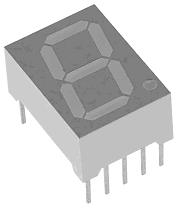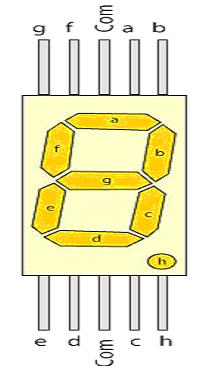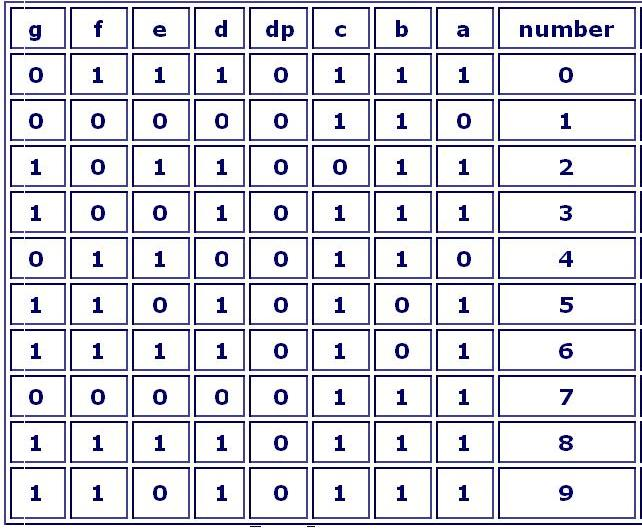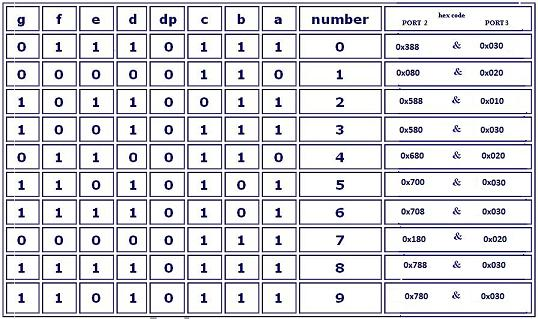Date: 28/05/2023

# Sample C-program/code for NXP LPCXpresso board

Display of numerics 0 to 9 on a 7-segment LED display

In this module a very-simple c program/code to display 0-9 on a 7-segment LED display using ARM Cortex M0 powered NXP's LPCXpresso board using 32 bit microcontroller (MCU) LPC1114F is explained.

Hardware requirements along with the board:

1. Seven segment display (Common Cathode)
2. 8-Resistors (3.3 Kilo ohms)
3. connecting wires
4. General purpose printed circuit board or a breadboard

Seven segment display:

Seven segment LED is device having seven light emitting diodes with either anode terminals (common anode ) or cathode terminals connected together to form a number '8' pattern as shown below in the picture.

To know more on 7-segment LED visit: http://ee.hawaii.edu/~sasaki/EE361/Fall06/Lab/7disp.html

and http://www.ee.ed.ac.uk/~gaa/DigilabNotes/Digilab/Components/node7.html

To use them you should know the pin configuration of the commercially available displays. As you must have guessed these displays should have nine pins( one for each segment + decimal point +common) but the available modules have two pins for common ground. They are internally connected. So they have total of 10 pins (see the picture below).

A 7-Segment display has 7-segments/pins named as a, b, c, b, e, f, g for forming the '8' pattern and and another segment/pin called 'h' for DP (decimal point) along with two extra pins for GND (in case of common cathode).To display numbers in seven-segment display, it is necessary to define the control signals

Below table shows the segment control for characters 0-9 as required for displaying numbers.Circuit diagram of 7-Segment Display Interfacing to ARM Cortex M0 microcontroller powered LPCXpresso board:

The below circuit connects 7-Segment display with port-2 and port-3 of microcontroller.Programming:
The sample c-program/code below continuously display digits 0-9 on seven-segment display and then repeating the sequence. The text below in dark-red color is the sample code:

#include "driver_config.h" //for including the header file
void delay() //delay function
{ int a;
for(a=0;a<3000000;a++);
}
int main ()
{
LPC_GPIO2->DIR=0x7C8; //Set the P2.10 ,P2.9 ,P2.8 ,P2.7, P2.6 ,P2.3 Pins as output pins
LPC_GPIO3->DIR=0x030; //set the P3.4 ,P3.5 pins as output pins.
while(1) //Infinite loop
{
// for 0
LPC_GPIO2->DATA=0x388 ; // (set the P2 bits as 0011 1000 1000)
LPC_GPIO3->DATA=0x030 ; // (set the P3 bits as 0000 0011 0000)
delay();

// for 1
LPC_GPIO2->DATA=0x080; // (set the P2 bits as 0000 1000 0000)
LPC_GPIO3->DATA=0x020; // (set the P3 bits as 0000 0010 0000)
delay();

// for 2

LPC_GPIO2->DATA=0x588; // (set the P2 bits as 0101 1000 1000)
LPC_GPIO3->DATA=0x010; // (set the P3 bits as 0000 0001 0000)
delay();

// for 3
LPC_GPIO2->DATA=0x580; // (set the P2 bits as 0101 1000 0000)
LPC_GPIO3->DATA=0x030; // (set the P3 bits as 0000 0011 0000)
delay();

//for 4
LPC_GPIO2->DATA=0x680; // set the P2 bits as 0110 1000 0000)
LPC_GPIO3->DATA=0x020; // (set the P3 bits as 0000 0010 0000)
delay();

//for 5
LPC_GPIO2->DATA=0x700; // (set the P2 bits as 0111 0000 0000)
LPC_GPIO3->DATA=0x030; // (set the P3 bits as 0000 0011 0000)
delay();

//for 6
LPC_GPIO2->DATA=0x708; // (set the P2 bits as 0111 0000 1000)
LPC_GPIO3->DATA=0x030; // (set the P3 bits as 0000 0011 0000)
delay();

//for 7
LPC_GPIO2->DATA=0x180; // (set the P2 bits as 0001 1000 0000)
LPC_GPIO3->DATA=0x020; // (set the P3 bits as 0000 0010 0000)
delay();

//for 8
LPC_GPIO2->DATA=0x788; // (set the P2 bits as 0111 1000 1000)
LPC_GPIO3->DATA=0x030; // set the P3 bits as 0000 0011 0000)
delay();

//for 9
LPC_GPIO2->DATA=0x780; // (set the P2 bits as 0111 1000 0000)
LPC_GPIO3->DATA=0x030; // (set the P3 bits as 0000 0011 0000)
delay();
}
return 0;
}

Please refer the table below for the hexa-decimal code required at the output port-2 and port-3 for displaying a particular number on the 7-segment.Click below to visit next and other embedded system
course modules:

Module 9: Controller Area Networking
(CAN)

Module 12: SPI Bus Interface

Module14: USB Interface

Module15: SRAM memory interface

Module16: Flash memory interface

Module17: LCD display panel interface

Module18: Touch pane interface

Module19: Audio/video interface

Previous Modules

Author: Srinivasa Reddy N